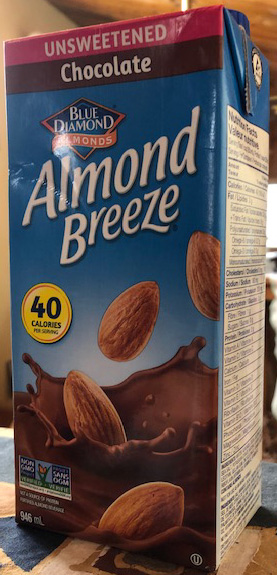SEARCH HOMEMath Central Quandaries & QueriesQuestion from Anjhelic: Karen is sipping juice from a 1 in. by 3 in. by 6 in tetra pack at the rate of 0.5in³/sec. How fast is the height of juice in the pack decreasing?Hi Anjhelic,

Here is an image of a Tetra Pak which has approximately the same dimensions as Karen's, a base of 1 in. by 3 in. and a height of 6in.The subject line of your email was Calculus but, because of the shape of the Tetra Pack, calculus is not needed. The cross section of the box is a 1 inch by 3 in rectangle. Every second the volume of juice removed is a rectangular slice of volume 0.5 cubic inches. The slice removed is 1 in. by 3 in. by its thickness. Thus

$1 \times 3 \times \mbox{ thickness } = \frac{1}{2} \mbox{ cubic inches.}$

Thus the slice removes every second is $\large \frac{1}{6}$ inches thick. Thus the height of the liquid in the box is decreasing at the rate of $\large \frac{1}{6}$ inches per second.

What about a calculus solution? Suppose that at tim $t$ seconds after Karen starts to drink and before the container is empty the volume of liquid in the box is $V(t)$ cubic inches and the height of the liquid in the box is $h(t)$ inches. Then we know that

$V(t) = 1 \times 3 \times h(t) = 3 h(t) \mbox{ cubic inches.}$

Differentiate each side by $t.$ You know that

$V^{\prime}(t) = \frac{1}{2} \mbox{ cubic inches per second.}$

Solve for $h^{\prime}(t).$

HarleyMath Central is supported by the University of Regina and The Pacific Institute for the Mathematical Sciences.